Ex 11.3

Chapter 11 Class 7 Exponents and Powers
Serial order wise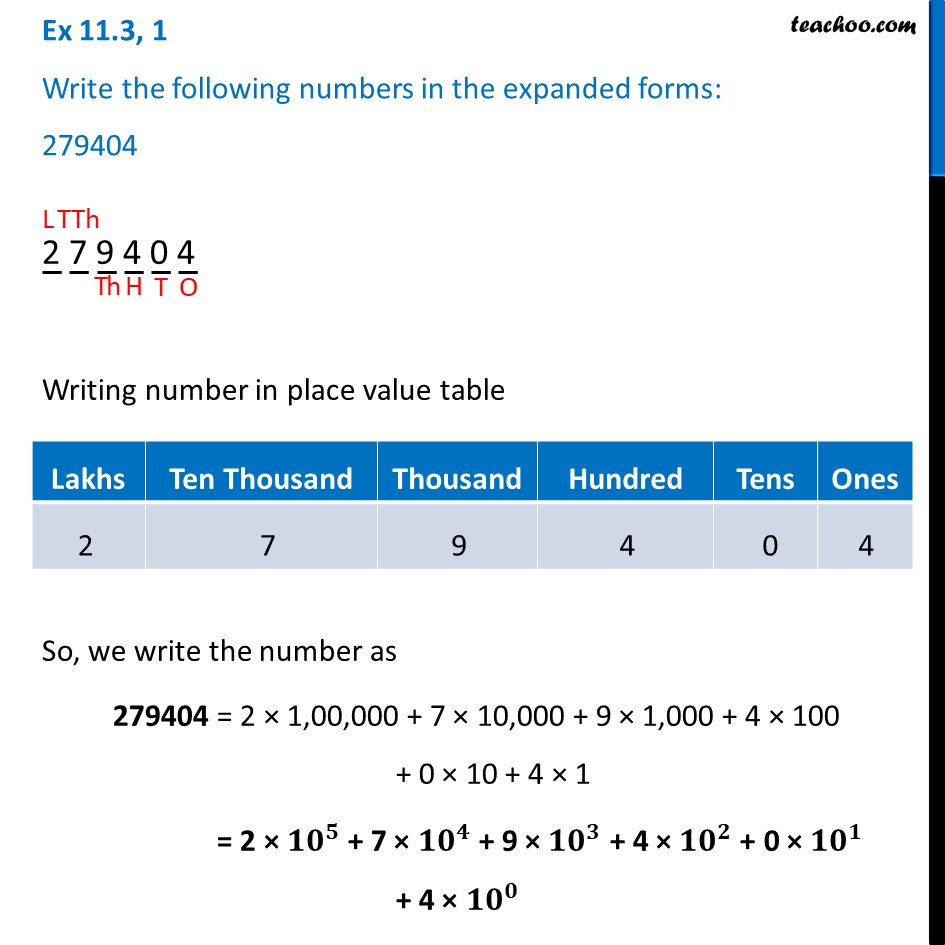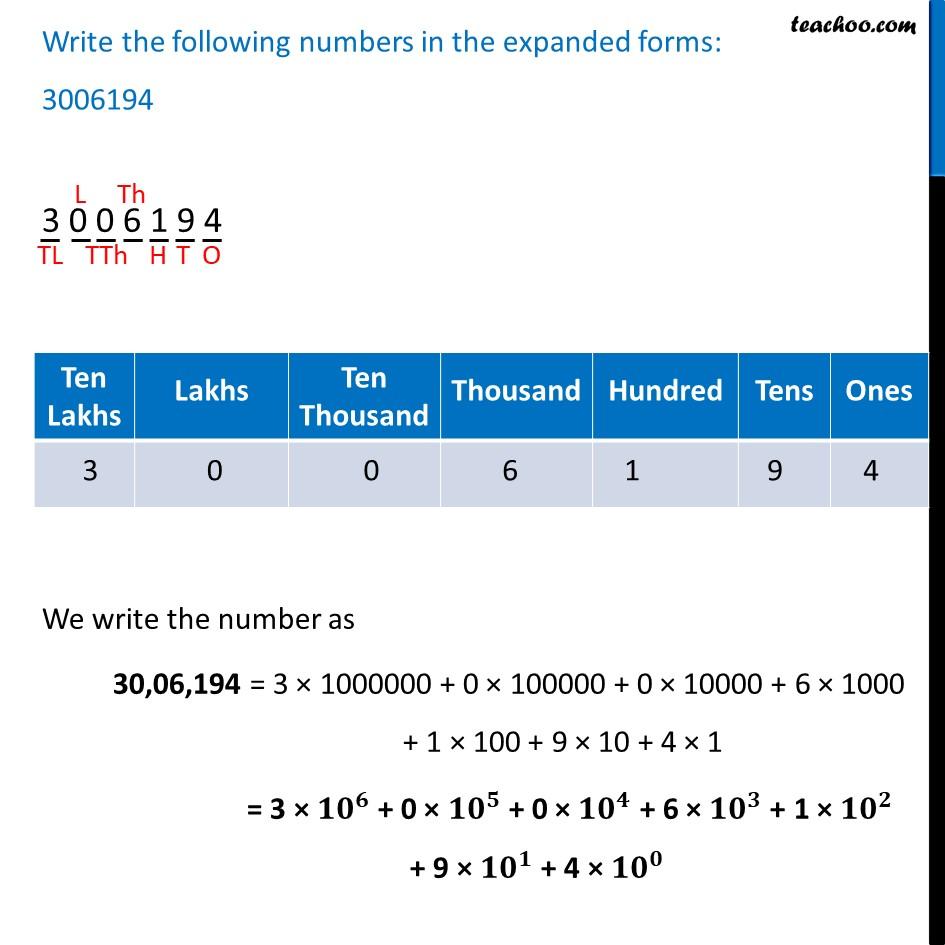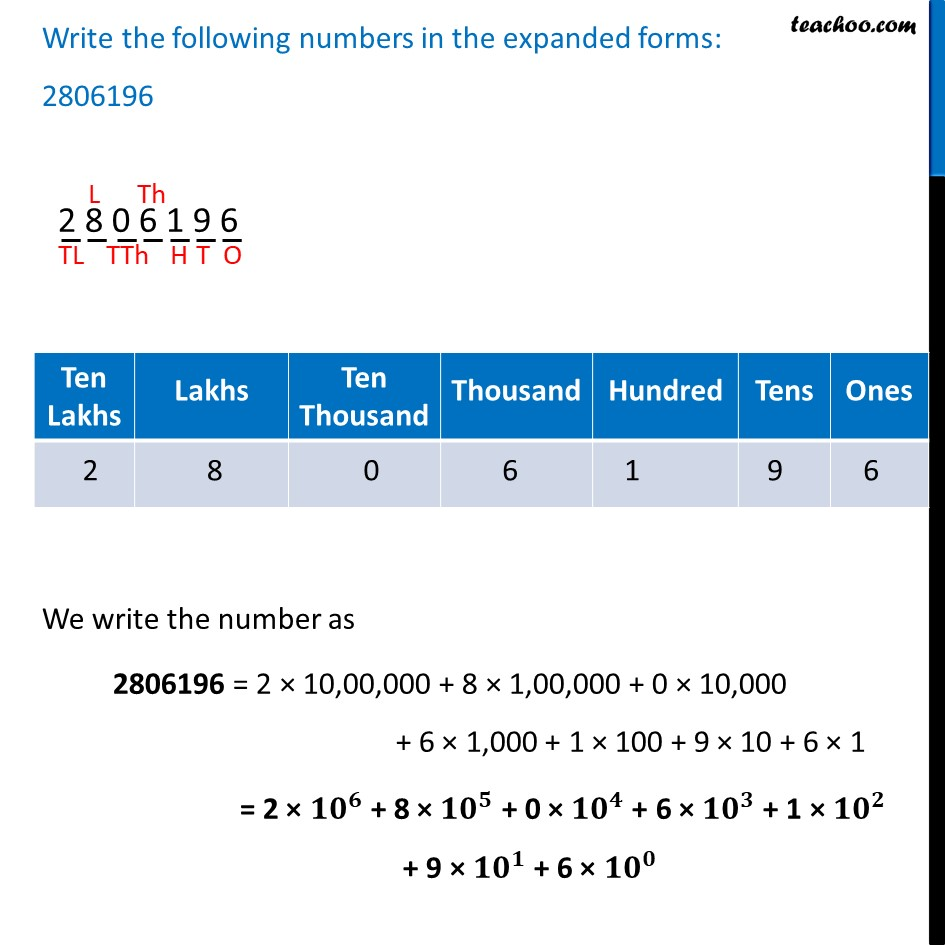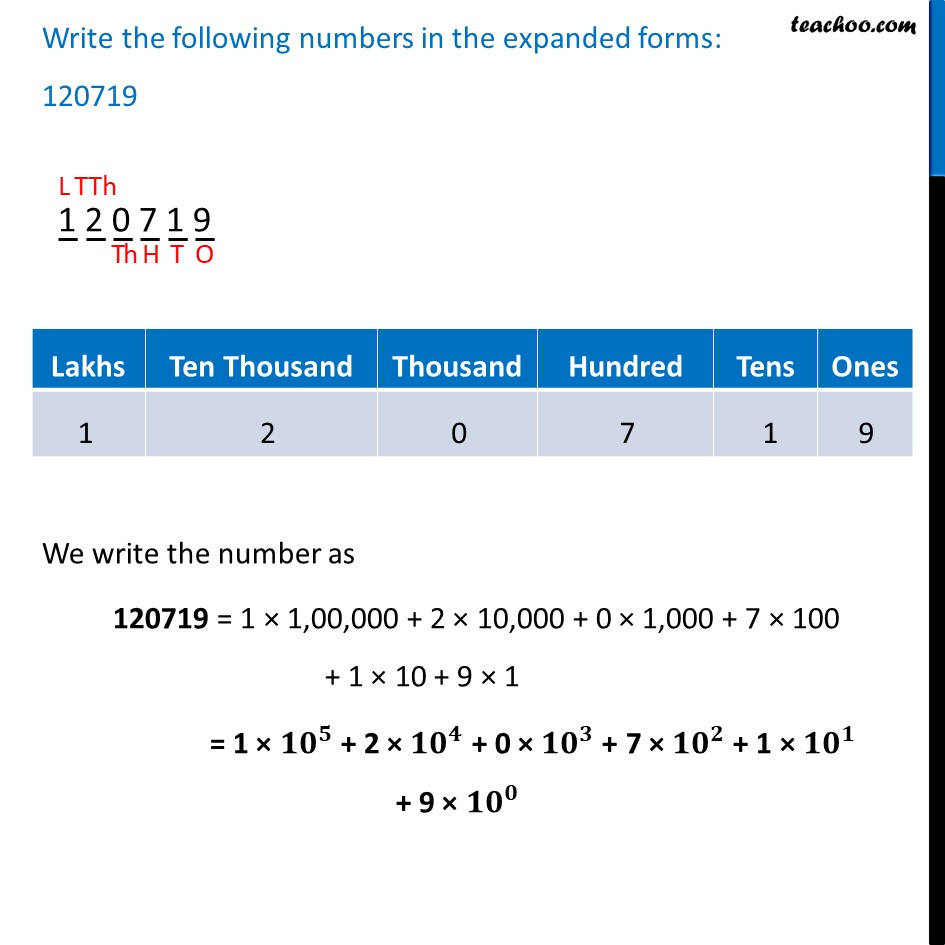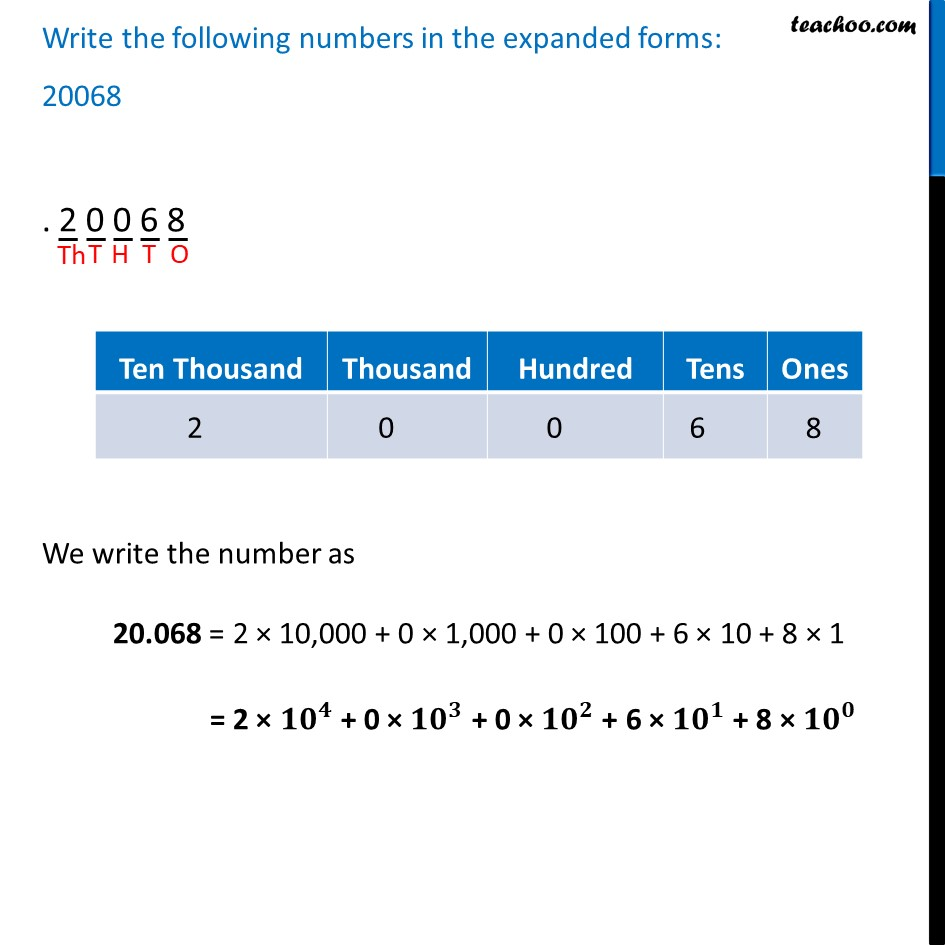Learn in your speed, with individual attention - Teachoo Maths 1-on-1 Class

### Transcript

Ex 11.3, 1 Write the following numbers in the expanded forms: 279404 279404 Writing number in place value table So, we write the number as 279404 = 2 × 1,00,000 + 7 × 10,000 + 9 × 1,000 + 4 × 100 + 0 × 10 + 4 × 1 = 2 × 〖10〗^5 + 7 × 〖10〗^4 + 9 × 〖10〗^3 + 4 × 〖10〗^2 + 0 × 〖10〗^1 + 4 × 〖10〗^0 Write the following numbers in the expanded forms: 3006194 3006194 We write the number as 30,06,194 = 3 × 1000000 + 0 × 100000 + 0 × 10000 + 6 × 1000 + 1 × 100 + 9 × 10 + 4 × 1 = 3 × 〖10〗^6 + 0 × 〖10〗^5 + 0 × 〖10〗^4 + 6 × 〖10〗^3 + 1 × 〖10〗^2 + 9 × 〖10〗^1 + 4 × 〖10〗^0 Write the following numbers in the expanded forms: 2806196 2806196 We write the number as 2806196 = 2 × 10,00,000 + 8 × 1,00,000 + 0 × 10,000 + 6 × 1,000 + 1 × 100 + 9 × 10 + 6 × 1 = 2 × 〖10〗^6 + 8 × 〖10〗^5 + 0 × 〖10〗^4 + 6 × 〖10〗^3 + 1 × 〖10〗^2 + 9 × 〖10〗^1 + 6 × 〖10〗^0 Write the following numbers in the expanded forms: 120719 120719 We write the number as .120719 = 1 × 1,00,000 + 2 × 10,000 + 0 × 1,000 + 7 × 100 + 1 × 10 + 9 × 1 = 1 × 〖10〗^5 + 2 × 〖10〗^4 + 0 × 〖10〗^3 + 7 × 〖10〗^2 + 1 × 〖10〗^1 + 9 × 〖10〗^0 Write the following numbers in the expanded forms: 20068 .20068 We write the number as 20.068 = 2 × 10,000 + 0 × 1,000 + 0 × 100 + 6 × 10 + 8 × 1 = 2 × 〖10〗^4 + 0 × 〖10〗^3 + 0 × 〖10〗^2 + 6 × 〖10〗^1 + 8 × 〖10〗^0